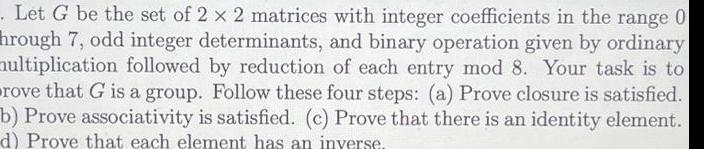Question:

# Let G be the set of 2 x 2 matrices with integer coefficients

Last updated: 9/14/2023Let G be the set of 2 x 2 matrices with integer coefficients in the range 0 hrough 7 odd integer determinants and binary operation given by ordinary multiplication followed by reduction of each entry mod 8 Your task is to rove that G is a group Follow these four steps a Prove closure is satisfied b Prove associativity is satisfied c Prove that there is an identity element d Prove that each element has an inverse